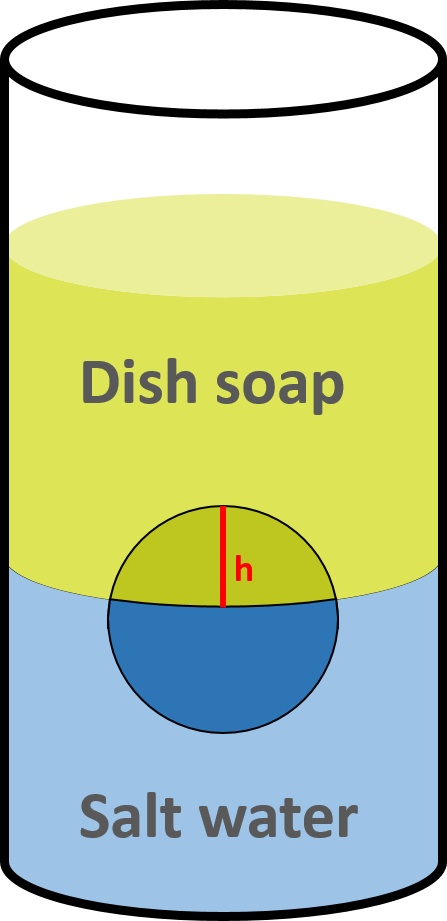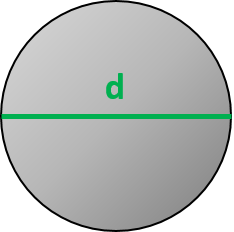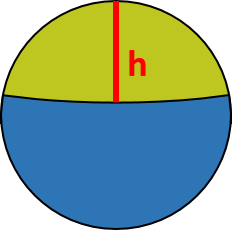# Buoyancy Experiment Calculator

Created by Wojciech Sas, PhD candidate
Reviewed by Steven Wooding
Last updated: Jan 11, 2021

Do you want to do buoyancy experiments at home? If so, here it is - the buoyancy experiment calculator.

Did you know how to measure the density of dish soap with saltwater and a golf ball? Read the article to find out what to prepare beforehand and how to perform the experiment. Then, use our tool to evaluate an unknown value (such as the density of dish soap). Experience the fun of physics for yourself!

## What is buoyancy? A brief recap

According to Archimedes' principle, if you put an object into some liquid, it exhibits an upward force FB which depends on the object's submerged part volume V and the liquid's density ρ. The denser the fluid, the greater the force:

FB = ρ * V * g.

where:

For simplicity, we assume that the buoyant force is one of two that affect the submerged body. The second one is the gravitational force. If our object is entirely submerged, it turns out that the relation between the buoyancy and the gravitational force is directly proportional to the relationship of their densities.

If the body is denser than the liquid, it sinks. If the object is less dense than the liquid, it floats.

The buoyant force also acts within the air (and other gases), but it's hardly noticeable in our everyday lives.

If you want to read more on the buoyant force, we have a dedicated buoyancy calculator for that!

## Buoyancy experiment preparation

We're going to estimate the density of dish soap. We propose the following set of items to do so:

• Dish soap;
• Water and salt to make saltwater;
• A golf ball;
• A measuring tape;
• A vessel to put our ball in; and
• A weight/scale.

Dish soap and saltwater are our two liquids. You are free to use any other liquids you want. Just make sure they are of different densities, and you know (or can easily calculate) one of them. Similarly, you can choose any other ball you can find. Ideally, it should float in one of the liquids and sink in the other.

Pour both liquids in the vessel, one after another, so that they don't mix. You should be able to see an interface between them. In the meantime, put a ball inside the vessel. It should float between liquids.

## Let's do the experiment! Step-by-step explanation

1. Evaluate the density of a ball:

• Weigh the ball and measure its diameter;
• Divide the mass by the volume to get the density.
2. Prepare a saltwater solution (we recommend to use salinities over 100‰ - aka 10%):

• For 100‰ solution, mix 100 g of salt and 900 g of pure water;
• For 200‰ solution, mix 200 g of salt and 800 g of pure water;
• For 300‰ solution, mix 300 g of salt and 700 g of pure water, and so on.
• Whenever you need more/less of the solution, you can simply use our proportion calculator.
• Estimate the density of the saltwater solution. One of the options is to choose one of the suggested salinities. An alternative is to use our water density calculator (go to its Advanced mode and type the mass of salt and water you used) and read saltwater density.
• If the solution's density is lower than the ball's density, try to make a higher salinity solution.1. Pour saltwater into the vessel.

2. Put the ball into the water. It should float.

3. SLOWLY pour the dish soap. Make sure that the two solutions do NOT mix! You should have two distinct layers with the ball in the middle.

4. Measure the height of that ball's part within the top solution, h.

5. Type all of the values into this buoyancy experiment calculator.

## How to calculate dish soap's density? Buoyancy experiment calculator in practice

1. Let's take a golf ball. The diameter equals 1.68", and its mass is 1.62 oz;

2. Work out the ball's density. It's circa 0.6525 oz/in³ or 1,129 kg/m³;

3. Prepare 20% saltwater solution by mixing 200 g of salt and 800 g of water;

4. Pour water into the vessel and throw a ball inside as well. Pour dish soap carefully;

5. Measure the height of the ball's cap. Let's say it's 0.5"; and

6. Voilà! The dish soap's density equals 0.5883 oz/in³ or 1,017.8 kg/m³ - that's our outcome.

If you're interested, check Advanced mode to see some additional information, like the contribution of each liquid to the total buoyant force.

What about trying with different liquids next? Let's go!

Although we can't assure your experiment's success, we do guarantee a lot of fun!

Wojciech Sas, PhD candidate
Ball's measurementsDiameter (d)
in
Mass (m)
oz
Density (ρ)
oz/cu in
First (bottom) liquid
Salt water (20% salinity)
💡 We can use precentages and per mille interchangeably. Salinity 20% is equivalent to 200‰, 25% is equivalent to 250‰, etc.
Density (ρ₁)
oz/cu in
Other measurementsHeight (h)
in
Second (top) liquid
Density (ρ₂)
oz/cu in
People also viewed…

### Diffraction grating

Diffraction grating calculator analyzes what happens when a light ray meets a surface with multiple apertures.

### Grams to cups

The grams to cups calculator converts between cups and grams. You can choose between 20 different popular kitchen ingredients or directly type in the product density.

### Korean age

If you're wondering what would your age be from a Korean perspective, use this Korean age calculator to find out.

### Projectile motion experiment

Blast off with this super fun projectile motion experiment calculator! Create a simple launcher and learn the basics of projectile motion.